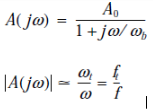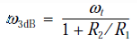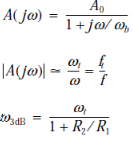Courses

# Test: Effect Of Finite Open-Loop Gain And Bandwidth On Circuit Performance

## 10 Questions MCQ Test Electronic Devices | Test: Effect Of Finite Open-Loop Gain And Bandwidth On Circuit Performance

Description
This mock test of Test: Effect Of Finite Open-Loop Gain And Bandwidth On Circuit Performance for Electrical Engineering (EE) helps you for every Electrical Engineering (EE) entrance exam. This contains 10 Multiple Choice Questions for Electrical Engineering (EE) Test: Effect Of Finite Open-Loop Gain And Bandwidth On Circuit Performance (mcq) to study with solutions a complete question bank. The solved questions answers in this Test: Effect Of Finite Open-Loop Gain And Bandwidth On Circuit Performance quiz give you a good mix of easy questions and tough questions. Electrical Engineering (EE) students definitely take this Test: Effect Of Finite Open-Loop Gain And Bandwidth On Circuit Performance exercise for a better result in the exam. You can find other Test: Effect Of Finite Open-Loop Gain And Bandwidth On Circuit Performance extra questions, long questions & short questions for Electrical Engineering (EE) on EduRev as well by searching above.
QUESTION: 1

### An internally compensated op amp is specified to have an open-loop dc gain of 106 dB and a unity gain bandwidth of 3 MHz. Find fb and the open-loop gain at fb.

Solution:

Use the equations below.QUESTION: 2

### A single-pole model has __________ db/decade roll-off of the gain.

Solution:

It is a standard characteristic of a single-pole model.

QUESTION: 3

### Single-pole model is also known as

Solution:

Single-pole model is also called dominant pole.

QUESTION: 4

An op amp having a 106-dB gain at dc and a single-pole frequency response with ft = 2 MHz is used to design a non-inverting amplifier with nominal dc gain of 100. The 3-dB frequency of the closed-loop gain is

Solution:

Use the equation below to obtain a frequency response curve and proceed further.QUESTION: 5

An internally compensated op amp has a dc open-loop gain of 106 V/V and an AC open-loop gain of 40 dB at 10 kHz. Estimate its gain–bandwidth product and its expected gain at 1 kHz.

Solution:

Use the following results.QUESTION: 6

An inverting amplifier with nominal gain of −20 V/V employs an op amp having a dc gain of 104 and a unity-gain frequency of 106 Hz. What is the 3-dB frequency f3dB of the closed-loop amplifier?

Solution:
QUESTION: 7

cascading two identical amplifier stages, each having a low-pass STC frequency response with a 3dB frequency f1, results in an overall amplifier with a 3dB frequency given by

Solution:
QUESTION: 8

Find the ft required for internally compensated op amps to be used in the implementation of the closed loop amplifiers with dc gain of +100 V/V and 3db bandwidth of 100kHz?

Solution:
QUESTION: 9

A particular op amp, characterized by a gain–bandwidth product of 20 MHz, is operated with a closed-loop gain of +100 V/V. What 3-dB bandwidth results? At what frequency does the closed-loop amplifier exhibit a −6° phase shift?

Solution:
QUESTION: 10

Find the ft required for internally compensated op amps to be used in the implementation of the closed loop amplifiers with dc gain of -2 V/V and 3db bandwidth of 10 MHz?

Solution:

-R2 ⁄ R1 = -2VV
f3db = 10 MHZ ft = 10 MHZ(2 + 1) = 30 MHZ.